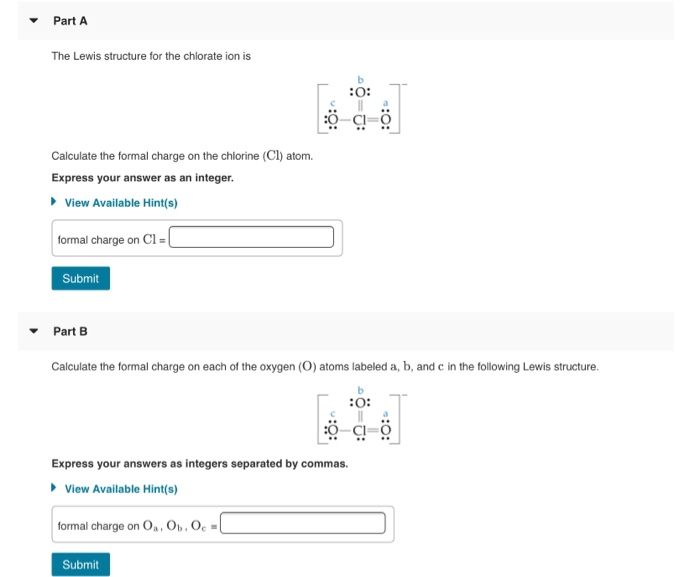# Calculate the formal charge on the chlorine (cl) atom.Part A The Lewis structure for the chlorate ion is :O: Calculate the formal charge on the chlorine (Cl) atom. Express your answer as an integer. View Available Hint(s) formal charge on CIEL Submit Part B Calculate the formal charge on each of the oxygen (O) atoms labeled a, b, and c in the following Lewis structure. 5 :0: 7 Express your answers as integers separated by commas. View Available Hint(s) formal charge on 0.0.0 Submit

A) Formal charge = valence electrons – lone pair electrons –
bonds
Formal charge = 7-2-5 = 0
B) Formal charge on Oa = 6-4-2 = 0
Formal charge on Ob = 6-4-2 = 0
Formal charge on Oc = 6-6-1 = -1
Oa,Ob,Oc = 0,0,1